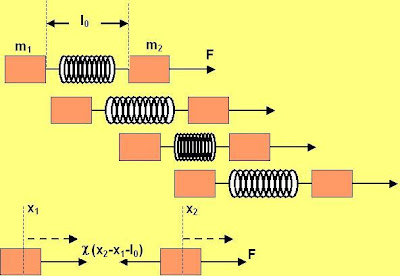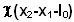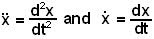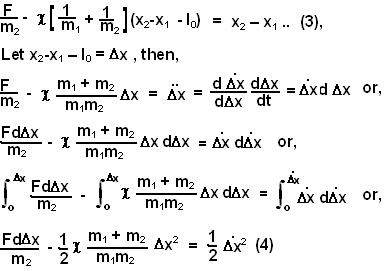## Saturday, May 10, 2008

### Irodov Solution 1.152As the force pulls m2, it stretches the spring and thus transmits the force to m1, pulling it too. The center of mass of the system acts like a point particle with mass (m1+m2) that is constantly pulled with a force F and so the center of mass simply accelerates at a constant rate F/(m1+m2) to the left.

With respect to an observer located on the center of mass of the system, he will see that the masses oscillate in simple harmonic motion. He will also feel as if a constant force (similar to force of gravity) of magnitude F is acting. So basically the whole system accelerates to the right while the mass keep oscillating about the center of mass as shown in the figure.

Suppose that the locations of the masses m1 and m2 are given by x1 and x2. There is only one force acting on m1 at any given point of time namely the force due to tension in the spring given by. Similarly on mass m2 there are two forces acting, i) the force F and ii) the tension in the spring. Hence we have,Here we use the dot notation,From (1) and (2) we can write,At the maximum and minimum stretched positions of the springand so for this condition in (4) we have two solutions,The corresponding total lengths of the spring will simply be.